[中文] Search Internet Search HelloDBA
Oracle Technology Site. email: fuyuncat@gmail.com  MSN: fuyuncat@hotmail.com# Oracle 12c Hybrid Histogram

[中文]

Author:  fuyuncat

Source:  www.HelloDBA.com

Date:  2013-08-27 04:18:04

Share to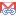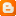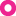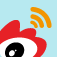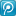In 12c, a new feature of the optimizer is that it provides new type histogram statistics data for the columns, TOP-N frequency histogram and HYBRID histogram. With them, the optimizer can choose a best execution plan more efficiently and accurately. Here I will talk about the Hybrid Histogram, how the statistics gather process generate it, and how it impact the optimizer to calculate the selectivity. <<<<<<<<<<<<<<<<<<<<<<<<<<<>>>>>>>>>>>>>>>>>>>>>>>>>>>>>>>>>>>>> A hybrid histogram combines characteristics of both height-based histograms and frequency histograms. This "best of both worlds" approach enables the optimizer to obtain better selectivity estimates in some situations. <<<<<<<<<<<<<<<<<<<<<<<<<<<>>>>>>>>>>>>>>>>>>>>>>>>>>>>>>>>>>>>>  The problem of Height-Balanced Histogram Shortly, the Hybrid Histogram is introduced to solve the inaccurate estimation issue of the Height-Balanced Histogram. First, let's review how selectivity for Height-Balanced Histogram is calculated:   If value is non-pop value,        selectivity=NewDensity                  =(total not null values row number - pop values rownum)/(number of non-pop values)/(total not null values row number)                  =((ssize-nv)-(ssize-nv)*PopBktCnt/BktCnt)/(NDV-PopValCnt)/(ssize-nv)                  =((ssize-nv)(1-*PopBktCnt/BktCnt))/(NDV-PopValCnt)/(ssize-nv)                  =((BktCnt-PopBktCnt)/BktCnt)/(NDV-PopValCnt);   If value is pop value and is not maximum value,        selectivity=PopBktNum/BktCnt;   If value is pop value and is also maximum value,        selectivity=(PopBktNum-0.5)/BktCnt;   While the procedure of generating Height-Balanced Histogram is simple.   * Assign the bucket size as the average row number of the distinct value; Put the distinct value into the buckets in sequence, and write down the cumulate size of the buckets and current distinct value row numbers;   * If free room of current bucket can contain the number of data of the distinct value, this distinct value will be put into the bucket, and continue to check the next value;   * If free room of current bucket can not contain the number of data of the distinct value, this value will be assigned to the next bucket. Meanwhile, if the gap between the cumulate size of the buckets and current distinct value row numbers larger than one bucket size, it will allocate more bucket to contain this value, and this value is considered as a popular value;   * For the values following the popular value, they will be assigned to a new bucket;   According to the calculation of the selectivity, it could be quite different for popular and non-popular values. While according to procedure of generating Height-Balanced Histogram, a popular value may just have a row number which is little bit larger than the bucket size, and it's considered as a popular value because the room of previous bucket is very little; and a non-popular value may have almost 2 bucket size row number, and it's not considered as a popular value because it has been assigned to empty bucket. The selectivity for such values will be far away from its real selectivity. Look at below example. SQL代码 HelloDBA.COM> truncate table oe.t_ntop;      Table truncated.      HelloDBA.COM> begin     2    for r in (select level lv from dual connect by level<=30) loop     3      insert into oe.t_ntop select r.lv, level from dual connect by level<=r.lv*10;     4    end loop;     5    commit;     6  end;     7  /      PL/SQL procedure successfully completed.      HelloDBA.COM>compute sum of cnt on dummy;   HelloDBA.COM>break on dummy;   HelloDBA.COM>select null dummy, a, count(a) cnt from oe.t_ntop group by a order by a;      D          A        CNT   - ---------- ----------              1         10              2         20              3         30   ... ...             26        260             27        270             28        280             29        290             30        300   *            ----------   s                  4650      30 rows selected.      HelloDBA.COM> exec dbms_stats.gather_table_stats(ownname=>'OE',tabname=>'T_NTOP',method_opt =>'for columns A size 25',estimate_percent => 100);      PL/SQL procedure successfully completed.      HelloDBA.COM>select endpoint_value, endpoint_number, nvl(endpoint_number - lag(endpoint_number,1) over (order by endpoint_value),1) num_in_bucket, endpoint_repeat_count from dba_histograms where owner='OE' and table_name = 'T_NTOP' and column_name = 'A';      ENDPOINT_VALUE ENDPOINT_NUMBER NUM_IN_BUCKET ENDPOINT_REPEAT_COUNT   -------------- --------------- ------------- ---------------------                1               0             1                     0                6               1             1                     0                9               2             1                     0               11               3             1                     0   ... ...               26              18             1                     0               27              20             2                     0               28              21             1                     0               29              23             2                     0               30              25             2                     0      22 rows selected.   Based on the gathered statistics data, we can calculate the NewDensity for non-popular values. SQL代码 HelloDBA.COM>select ((BktCnt-PopBktCnt)/BktCnt)/(NDV-PopValCnt) NewDensity     2  from (select sample_size ssize, num_nulls nv, num_distinct ndv, num_buckets BktCnt, density OldDensity     3        from dba_tab_col_statistics     4        where owner='OE' and table_name = 'T_NTOP' and column_name = 'A'),     5        (select count(num_in_bucket) PopValCnt, sum(num_in_bucket) PopBktCnt     6         from (select endpoint_value, endpoint_number, endpoint_number - lag(endpoint_number,1) over (order by endpoint_value) num_in_bucket     7               from dba_histograms     8               where owner='OE' and table_name = 'T_NTOP' and column_name = 'A')     9         where num_in_bucket>1);      NEWDENSITY   ----------   .026153846   According to the histogram, 27 is a popular value, 28 is a non-popular data. We can manually estimate the rows after filtered by matching these 2 values, SQL代码 HelloDBA.COM>select 4650*2/25 CAL_ROWS_OF_27, 4650*0.026153846 CAL_ROWS_OF_28 from dual;      CAL_ROWS_OF_27 CAL_ROWS_OF_28   -------------- --------------              372     121.615384   And let's check the optimizer estimate result. SQL代码 HelloDBA.COM>set autot trace exp   HelloDBA.COM>select * from oe.t_ntop where a=27;      Execution Plan   ----------------------------------------------------------   Plan hash value: 1181773393      ----------------------------------------------------------------------------   | Id  | Operation         | Name   | Rows  | Bytes | Cost (%CPU)| Time     |   ----------------------------------------------------------------------------   |   0 | SELECT STATEMENT  |        |   372 |  2232 |     5   (0)| 00:00:01 |   |*  1 |  TABLE ACCESS FULL| T_NTOP |   372 |  2232 |     5   (0)| 00:00:01 |   ----------------------------------------------------------------------------      Predicate Information (identified by operation id):   ---------------------------------------------------         1 - filter("A"=27)      HelloDBA.COM>select * from oe.t_ntop where a=28;      Execution Plan   ----------------------------------------------------------   Plan hash value: 1181773393      ----------------------------------------------------------------------------   | Id  | Operation         | Name   | Rows  | Bytes | Cost (%CPU)| Time     |   ----------------------------------------------------------------------------   |   0 | SELECT STATEMENT  |        |   122 |   732 |     5   (0)| 00:00:01 |   |*  1 |  TABLE ACCESS FULL| T_NTOP |   122 |   732 |     5   (0)| 00:00:01 |   ----------------------------------------------------------------------------      Predicate Information (identified by operation id):   ---------------------------------------------------         1 - filter("A"=28)   The optimizer estimated results match the calculated ones. However, these values are quite different from their real values (270, 280) considering the non-null value row number. Especially, the really size of 27 and 28 are actually very close, but there is a huge gap between their selectivity. This could lead the optimizer choose a bad performance execution plan. How Hybrid Histogram solve this problem The Hybrid Histogram will not simply use the Endpoint Number to indicate the endpoint value is a popular value and to represent its ratio. Instead, it has an additional information (a new column in the histogram table, endpoint_repeat_count) to store the size of the endpoint value of the bucket. Therefore, selectivity of endpoint value in Hybrid Histogram will be much more accurate than endpoint value in height balanced histogram. In Hybrid Histogram, a value is considered as a popular value when it has more number of data than the average bucket size (not null value row number/bucket number). The calculation of selectivity in Hybrid Histogram is,   if the value is a popular value (popular value will always be the endpoint value), the selectivity will be endpoint_repeat_count/(not null value row number);     selectivity = endpoint_repeat_count/(not null value row number)   if the value is a non-popular value and is not the endpoint value, the selectivity will be the new density calculated from non-popular data. The formula of the new density is same as the one of Height Balanced Histogram, but the elements of the formula have different value;     selectivity = NewDensity = ((BktCnt-PopBktCnt)/BktCnt)/(NDV-PopValCnt)     Those *Cnt are actually the sum data of the specified kinds of value, instead of count of the specified value   if the value is a non-popular value and is the endpoint value, the selectivity depends on the ratio of its endpoint_repeat_count in non-null value number and the new density. In a word, the optimizer will choose the largest one as its selectivity;     selectivity = Greatest(NewDensity, endpoint_repeat_count/(not null value row number))   Take an example to be looked into, SQL代码 HelloDBA.COM>exec dbms_stats.gather_table_stats(ownname=>'OE',tabname=>'T_NTOP',method_opt =>'for columns A size 25');      PL/SQL procedure successfully completed.      HelloDBA.COM>select endpoint_value, endpoint_number, nvl(endpoint_number - lag(endpoint_number,1) over (order by endpoint_value), endpoint_number) bucket_size, endpoint_repeat_count from dba_histograms where owner='OE' and table_name = 'T_NTOP' and column_name = 'A';      ENDPOINT_VALUE ENDPOINT_NUMBER BUCKET_SIZE ENDPOINT_REPEAT_COUNT   -------------- --------------- ----------- ---------------------                1              10          10                    10                6             210         200                    60                7             280          70                    70   ... ...               28            4060         280                   280               30            4650         590                   300      25 rows selected.      HelloDBA.COM>select ((BktCnt-PopBktCnt)/BktCnt)/(NDV-PopValCnt) NewDensity, pop_threshold     2  from (select count(1) PopValCnt, sum(endpoint_repeat_count) PopBktCnt, ndv, BktCnt, pop_threshold     3          from (select (sample_size - num_nulls) BktCnt, num_distinct ndv, num_buckets, density OldDensity, (sample_size-num_nulls)/num_buckets pop_threshold     4                from dba_tab_col_statistics     5                where owner='OE' and table_name = 'T_NTOP' and column_name = 'A'),     6               dba_histograms     7         where owner='OE' and table_name = 'T_NTOP' and column_name = 'A'     8         and endpoint_repeat_count>pop_threshold     9         group by ndv, BktCnt, pop_threshold);      NEWDENSITY POP_THRESHOLD   ---------- -------------   .022637238           186   We choose 3 values to check their selectivity:  SQL代码 HelloDBA.COM>set autot trace exp   HelloDBA.COM>select * from oe.t_ntop where a=29;      Execution Plan   ----------------------------------------------------------   Plan hash value: 1181773393      ----------------------------------------------------------------------------   | Id  | Operation         | Name   | Rows  | Bytes | Cost (%CPU)| Time     |   ----------------------------------------------------------------------------   |   0 | SELECT STATEMENT  |        |   105 |   630 |     5   (0)| 00:00:01 |   |*  1 |  TABLE ACCESS FULL| T_NTOP |   105 |   630 |     5   (0)| 00:00:01 |   ----------------------------------------------------------------------------      Predicate Information (identified by operation id):   ---------------------------------------------------         1 - filter("A"=29)   29 is not a endpoint value, so, it's not considered as a popular value although its size larger than threshold --- this is a defect of Hybrid Histogram, it will only happen in the last bucket. It's selectivity equal to the new density, and the filtered row number is trunc(4650*.022637238)=105; SQL代码 HelloDBA.COM>select * from oe.t_ntop where a=30;      Execution Plan   ----------------------------------------------------------   Plan hash value: 1181773393      ----------------------------------------------------------------------------   | Id  | Operation         | Name   | Rows  | Bytes | Cost (%CPU)| Time     |   ----------------------------------------------------------------------------   |   0 | SELECT STATEMENT  |        |   300 |  1800 |     5   (0)| 00:00:01 |   |*  1 |  TABLE ACCESS FULL| T_NTOP |   300 |  1800 |     5   (0)| 00:00:01 |   ----------------------------------------------------------------------------      Predicate Information (identified by operation id):   ---------------------------------------------------         1 - filter("A"=30)   This is a popular value. It's selectivity equal is endpoint_repeat_count/(not null value row number), and the filtered row number is trunc(4650*300/4650)=300; SQL代码 HelloDBA.COM>select * from oe.t_ntop where a=11;      Execution Plan   ----------------------------------------------------------   Plan hash value: 1181773393      ----------------------------------------------------------------------------   | Id  | Operation         | Name   | Rows  | Bytes | Cost (%CPU)| Time     |   ----------------------------------------------------------------------------   |   0 | SELECT STATEMENT  |        |   110 |   660 |     5   (0)| 00:00:01 |   |*  1 |  TABLE ACCESS FULL| T_NTOP |   110 |   660 |     5   (0)| 00:00:01 |   ----------------------------------------------------------------------------      Predicate Information (identified by operation id):   ---------------------------------------------------         1 - filter("A"=11)   This is not a popular value but a endpoint value. It's selectivity is the maximum value of the new density and endpoint_repeat_count/(not null value row number), and the filtered row number is trunc(4650*greatest(0.022637238,110/4650))=110; In Hybrid Histogram, most of values have a more accurate selectivity than in Height Balanced Histogram. How Hybrid Histogram is generated To get a Hybrid Histogram, 3 conditions should be fulfilled. <<<<<<<<<<<<<<<<<<<<<<<<<<<>>>>>>>>>>>>>>>>>>>>>>>>>>>>>>>>>>>>> Starting in Oracle Database 12c Release 1 (12.1), the database creates hybrid histograms when all of the following conditions are true:   n is less than the NDV, where n is the user-specified number of buckets. If no number is specified, then n defaults to 254.   The criteria for top frequency histograms do not apply. See "Criteria For Frequency Histograms."   The sampling percentage is AUTO_SAMPLE_SIZE If users specify their own percentage, then the database creates frequency or height-balanced histograms. <<<<<<<<<<<<<<<<<<<<<<<<<<<>>>>>>>>>>>>>>>>>>>>>>>>>>>>>>>>>>>>> Note: For the sampling percentage, it can also be assigned as NULL (means computed by the statistics gathering process) to generate Hybrid Histogram. And since the Hybrid Histogram to solve the problem of Height-Balanced, they are mutually exclusive. That is to say, if the condition of Hybrid Histogram is fulfilled, the statistics data gathering process will not try Height-Balanced. There are 2 ways to generate a hybrid histogram,   * When sampling percentage is NULL (computed by the process), the gathering process will use a query to get the raw data of distinct values, and then fill them into bucket in sequence. The query to get the raw data looks like below.     select substrb(dump(val,16,0,64),1,240) ep,  freq, cdn, ndv, (sum(pop) over()) popcnt,  (sum(pop*freq) over()) popfreq,  substrb(dump(max(val) over(),16,0,64),1,240) maxval,  substrb(dump(min(val) over(),16,0,64),1,240) minval  from (select val, freq,  (sum(freq) over()) cdn, (count(*) over()) ndv,  (case when freq > ((sum(freq) over())/25)  then 1  else 0 end) pop from  (select /*+  no_parallel(t) no_parallel_index(t) dbms_stats cursor_sharing_exact use_weak_name_resl dynamic_sampling(0) no_monitoring xmlindex_sel_idx_tbl no_substrb_pad  */ "A"  val, count("A") freq  from "OE"."T_NTOP" t  where "A" is not null  group by "A")) order by val;   * When sampling percentage is AUTO_SAMPLE_SIZE, the gathering process will try Frequency Histogram first, and if the condition of adopting Frequency Histogram is failed, e.g. MNB < NDV, the process will convert those data entries gathered for Frequency Histogram to Hybrid Histogram;   Similar to height balanced histogram, the data entries will be filled into the bucket with a average size (not-null value number/bucket number, which is also the popular value threshold) in sequence. Once spilled, the next entry will be put into the next bucket. The entry size of the last entry in the bucket will be stored as the endpoint repeat count. For the last bucket, it will contain all of entries not assigned yet, no matter the non-endpoint value is popular value or not. --- Fuyuncat ---